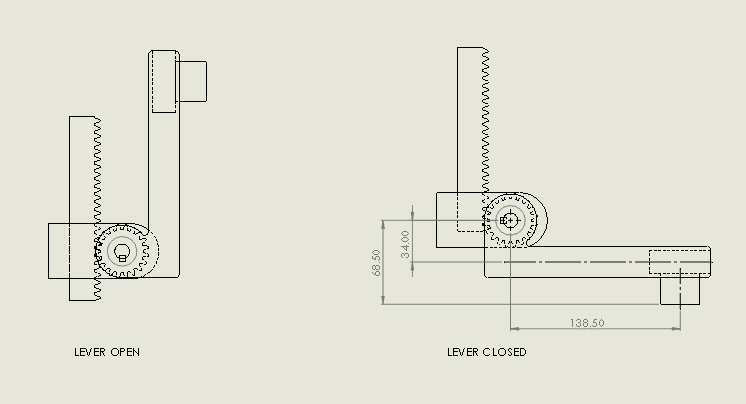# Lever Torque Calculation

So..

I'm trying to figure out if a design Idea has any potential. The figure below shows a lever mechanism operated by a rack and pinion gear assembly. I've calculated the torque in the pinion to be 252 Nm.

What will be the force at the contact pad, generated by the lever when in the closed position?#### Attachments

JBA
Gold Member
The resulting force is equal to the 252 Nm torque x the 138.5 mm? length (converted to m) in your drawing. (Note: No drawing is complete without at least a note specifying the units of its dimensions.)

•IanPibworth and berkeman
Oh.. its really as simple as that? I feel a bit stupid asking now, I thought because of the offset there would be an additional calculation.

Yes, the full drawing border has the specified units, in this case its mm.

CWatters
Homework Helper
Gold Member
The resulting force is equal to the 252 Nm torque x the 138.5 mm? length (converted to m) in your drawing. (Note: No drawing is complete without at least a note specifying the units of its dimensions.)
I think that should be

Force = torque/distance = 252/0.138

JBA
Gold Member
{/QUOTE] JBA, post: 6048274, member: 570081"]The resulting force is equal to the 252 Nm torque x the 138.5 mm? length (converted to m)[/QUOTE]

I intended the conversion to refer to the 138.5 mm length; but, I can see how it might be a bit ambiguous and misconstrued as referring to the multiplication result.

CWatters
Homework Helper
Gold Member
I wasn't referring to the conversion...

Torque = force * distance
so
Force = torque / distance

JBA